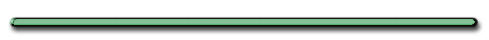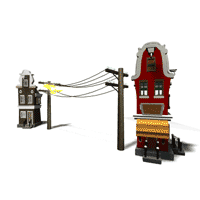HomeFlowers in Liquid Nitrogen

Energy
- Capacity to do work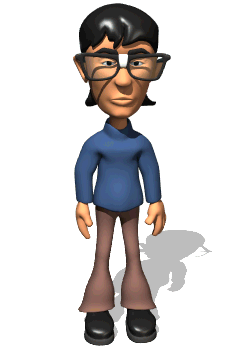Light can be converted to heat energy which can apply a force and make something move.Gasoline is a form of energy
because it can do work.

Car Engine

by Don IonLevers give you a 'mechanical advantage'

[ Flash ]Work always involves some tradeoff. Name 2 ways 1st gear is different than 10th gear.1st gear requires

a small force and a lot pedaling,

while 10th gear requires

little pedaling but  larger force.

An Incline Plane is a Simple Machine

[ Flash ]

I) Work - when a force causes an object to move in the direction of that force.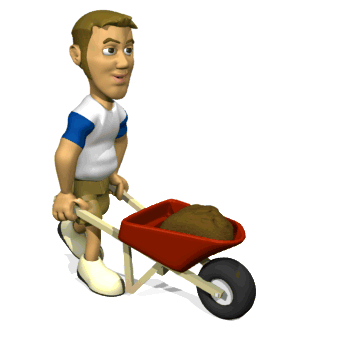Work and Energy

**Scalar**

(means direction doesn't count)

1 + 1 always  = 2

A) Equation

 W = F Δd (Nm) (N) (m)

F - force acting in
same direction as motion

Δd - displacement

Other units for work (Joule) or Kg m2/sec2Challenge Question: Two people exert a 10 Newton force on a block for 2 meters.  One person exerts her force at a 50-degree angle.

 Person 1 Person 2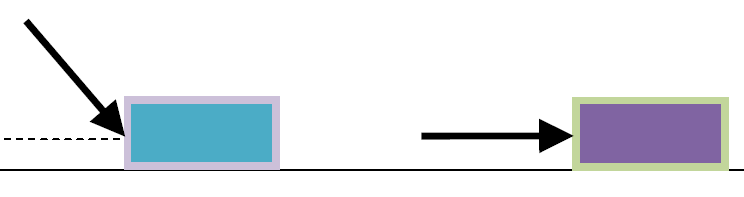Did both people do the same work???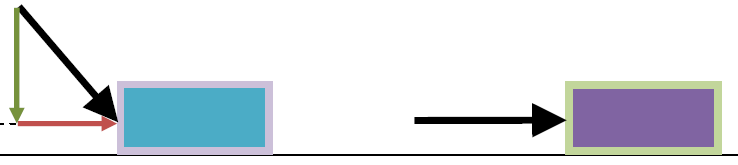Person 2 does more work
All of her force goes to
moving the block"Do the young man's HANDS do any work on the books he's holding?""No. The books do not move in the direction of the hand's force. (up) His legs do the work"Compound pulleys reduce the force
needed to do work."What distance would the rope have to be pulled to make the weight rise 1 m ?"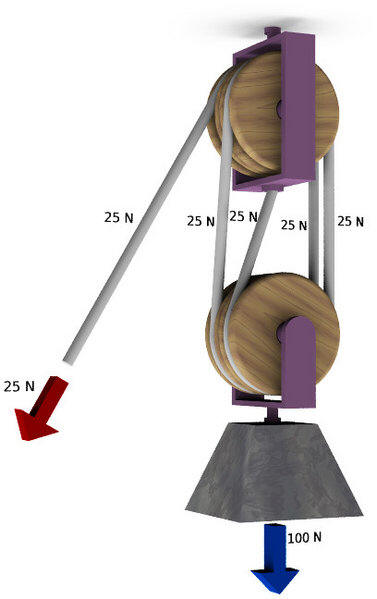(Borrar todas) (act) 09:40 7 ago 2004 (Ilustración de un polispasto de cuatro poleas.)

 W = F Δd (Nm) (N) (m)"To lift the weight 1 m the rope has to be pulled 4 m.  F reduced by 1/4, d increased by 4 times"

Machines reduce force but
increase the distance
the force is applied
by an equal magnitude.

 Ex) A 20.0-N force is used to push a 2.00-kg cart a distance of 5.00 m. Work done on cart?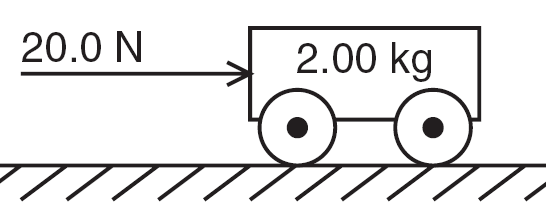a)150. J  b)100. J c)200. J d)40.0 J

Ex) A 20.0-N force is used to push a 2.00-kg cart a distance of 5.00 m. Work done on cart =W = F Δd (Nm) (N) (m)

a)150.J b)100. J c)200.J d)40.0 JB) Work and F vs. d diagram (plot)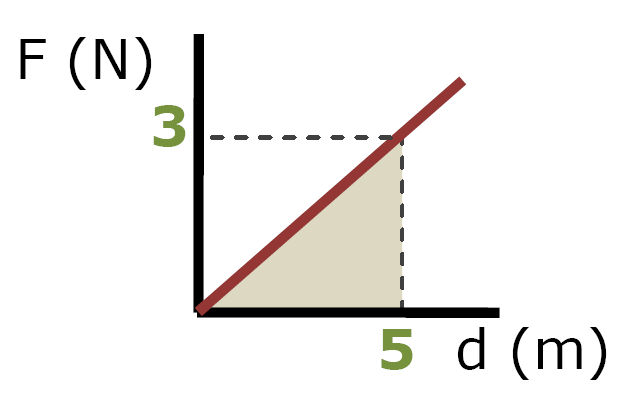Area under a Force vs. d plot = Work

W = Area Under Plot

= 1/2bh

= 1/2(5 m)(3 N)

7.5 NmRamps reduce the force needed to move
a body upward against the force of gravity."If AB/BC = 3, what is the smallest mass ball P could have?" (assume no friction)

If W = 12 N, P could be as small as ....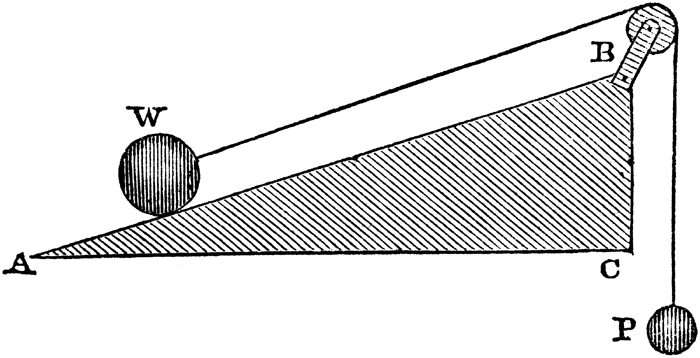J. L. Comstock A System of Natural Philosophy: Principles of Mechanics
(: Pratt, Woodford, and Company, 1850) 92

Clipart ETC"Ball P could be 1/3 the mass of ball W" 4 N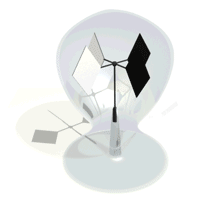Potential Energy